Homework Help Question & Answers

# It’s review question, I need this as soon as possible. Thank you 3) For thè diferential equation: (a) The point zo =-1 is an ordinary point. Compute the recursion formula for the coefficients of...It’s review question, I need this as soon as possible. Thank you

3) For thè diferential equation: (a) The point zo =-1 is an ordinary point. Compute the recursion formula for the coefficients of the power series solution centered at zo- -1 and use it to compute the first three nonzero terms of the power series when -1)-s and v(-1)-0. (25 points) (b) The point z = 0 is a regular singular point . Compute the associated Euler equation and compute the recursion formula for the coefficients of the series solution centered at zo = 0 which is associated with the smaller root. (20 points)

3) For thè diferential equation: (a) The point zo =-1 is an ordinary point. Compute the recursion formula for the coefficients of the power series solution centered at zo- -1 and use it to compute the first three nonzero terms of the power series when -1)-s and v(-1)-0. (25 points) (b) The point z = 0 is a regular singular point . Compute the associated Euler equation and compute the recursion formula for the coefficients of the series solution centered at zo = 0 which is associated with the smaller root. (20 points)

#### Homework Answers

Answer #1 ✔ Recommended Answer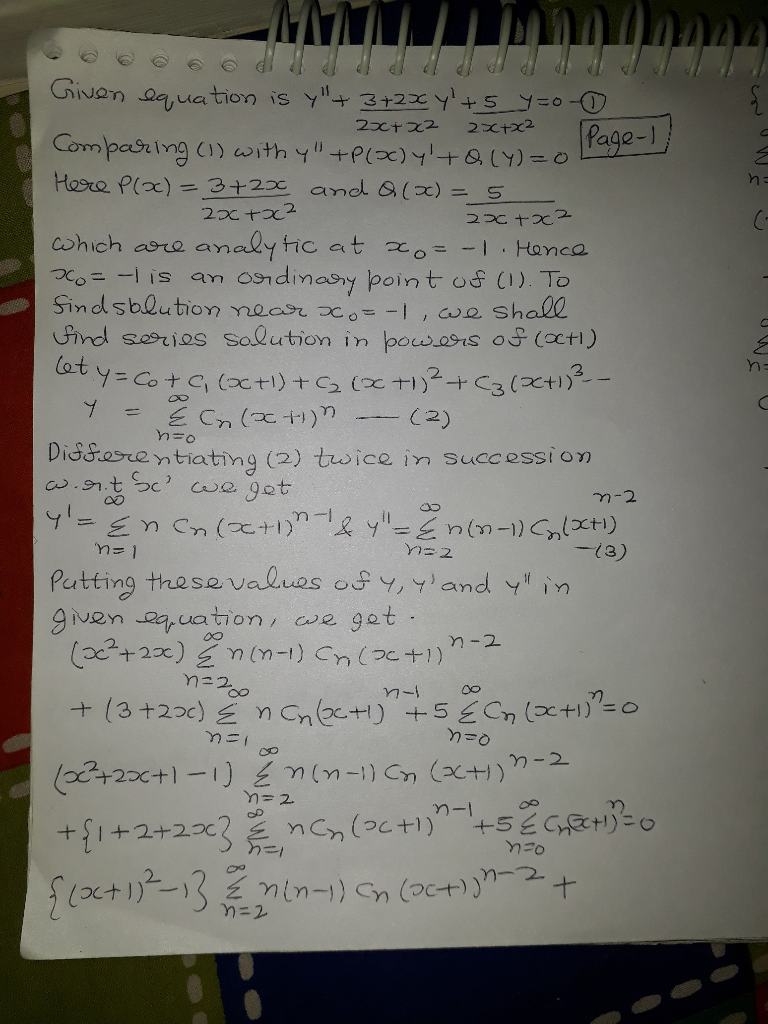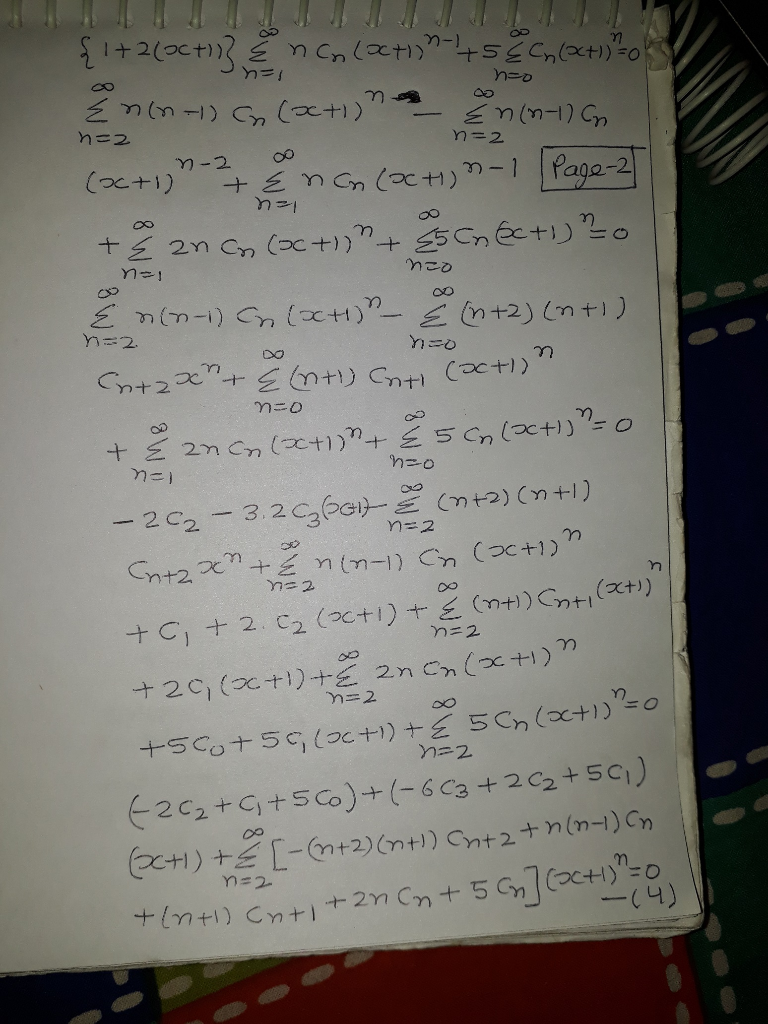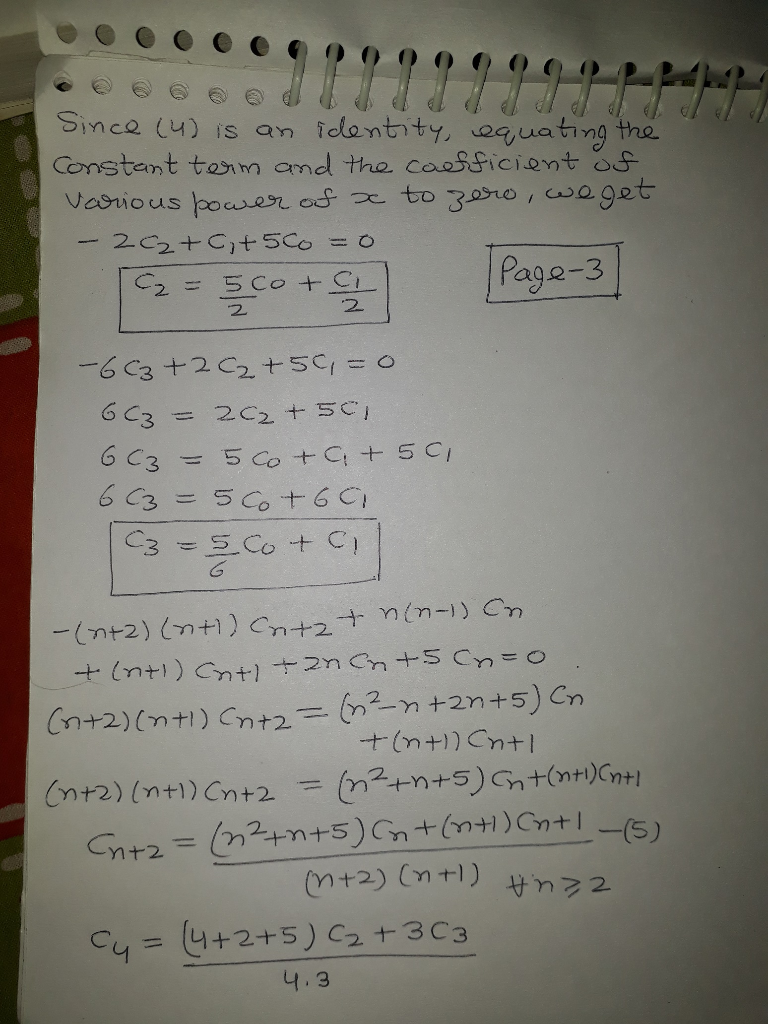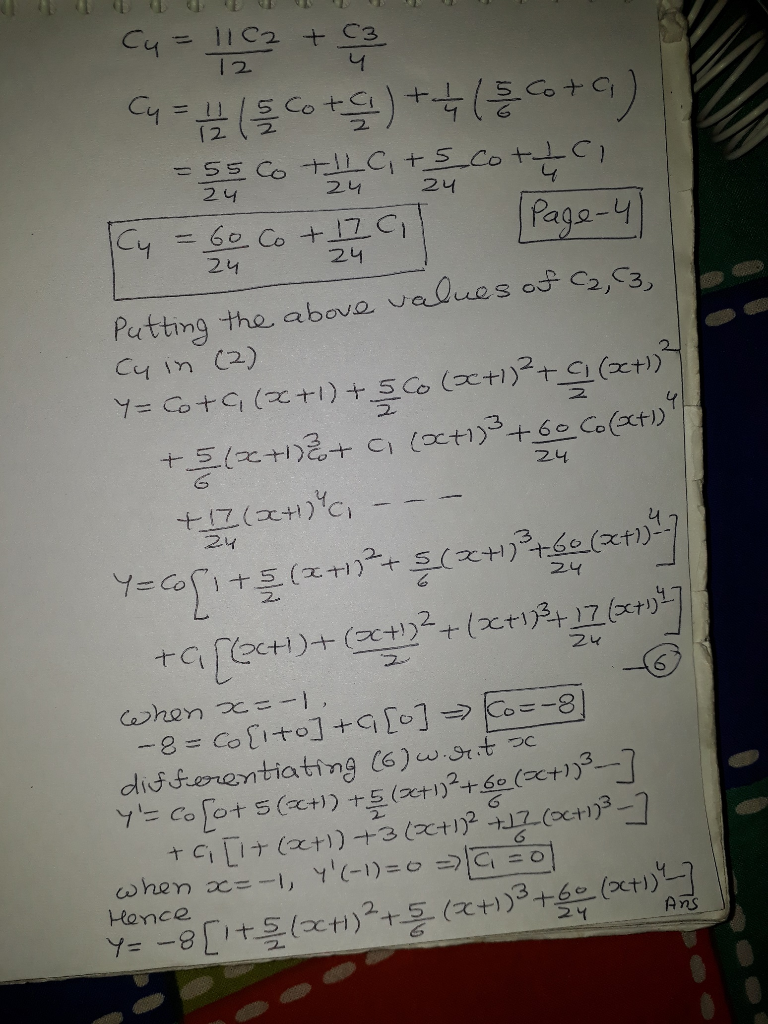Add a comment
Answer #2
[REMOVED]
Know the answer?
Your Answer:

#### Post as a guest

Your Name:

What's your source?

#### Earn Coin

Coins can be redeemed for fabulous gifts.

Not the answer you're looking for? Ask your own homework help question. Our experts will answer your question WITHIN MINUTES for Free.
Similar Homework Help Questions
• ### B only It’s review question, I need this as soon as possible. Thank you 3) Pol the diferential equation: (a) The point o -1 is an ordinary point. Compute the recursion formula for the coeffici...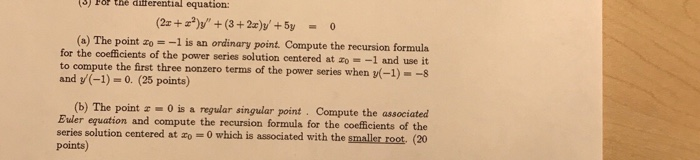B only It’s review question, I need this as soon as possible. Thank you 3) Pol the diferential equation: (a) The point o -1 is an ordinary point. Compute the recursion formula for the coefficients of the power series solution centered at zo- -1 and use it to compute the first three nonzero terms of the power series when (-1)--s and y(-1)-0. (25 points) (b) The point 0 is a regular singular point Compute the associated Buler equation and compute...

• ### 3) Pol the diferential equation: (a) The point o -1 is an ordinary point. Compute the recursion formula for the coefficients of the power series solution centered at zo- -1 and use it to compute...3) Pol the diferential equation: (a) The point o -1 is an ordinary point. Compute the recursion formula for the coefficients of the power series solution centered at zo- -1 and use it to compute the first three nonzero terms of the power series when (-1)--s and y(-1)-0. (25 points) (b) The point 0 is a regular singular point Compute the associated Buler equation and compute the recursion formula for the coefficients of the series solution centered at o 0...

• ### (6) (18 points) For the differential equation: Compute the recursion formula for the coefficients...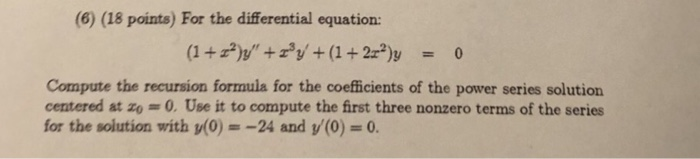(6) (18 points) For the differential equation: Compute the recursion formula for the coefficients of the power series solution centered at zo 0.Use it to compute the first three nonzero terms of the series for the solution with y(0) =-24 and y(0) = 0. (6) (18 points) For the differential equation: Compute the recursion formula for the coefficients of the power series solution centered at zo 0.Use it to compute the first three nonzero terms of the series for the...

• ### (4) (12 points)For the differential equation: Compute the recursion formula for the coefficients of the power series solution centered at o 0 and use it to compute the first three nonzero terms of...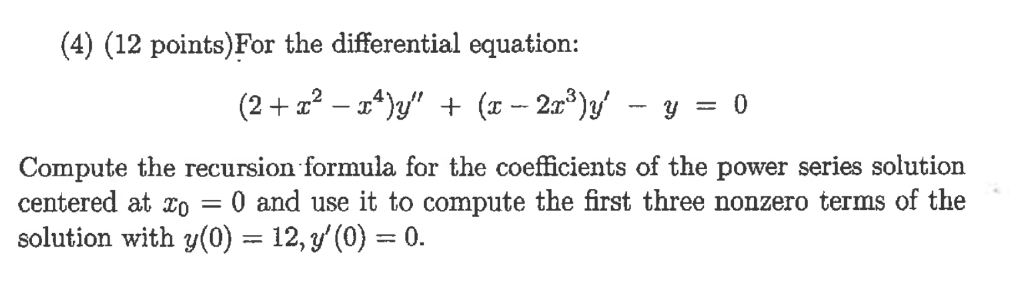(4) (12 points)For the differential equation: Compute the recursion formula for the coefficients of the power series solution centered at o 0 and use it to compute the first three nonzero terms of the solution with y(0) 12, y'(0)0. (5) (12 points)For the equation y" - 5ty -7y 0 (t>0), (t)t is a solution (a) Use the method of Reduction of Order to obtain a second, independent solution. (b) Solve the equation directly, using that it is an Euler Equation....

• ### 1) Show that zo-0 is a regular singular point for the diferenta equation Zo = 0 is a regular sing...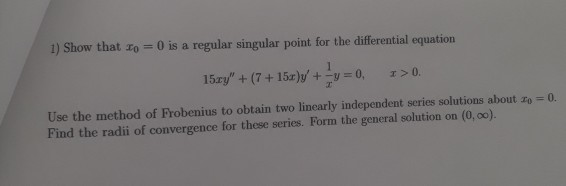please show the recurrence formula 1) Show that zo-0 is a regular singular point for the diferenta equation Zo = 0 is a regular singular point for the differential equation 15ェy" + (7 + 15r)y, +-y = 0, x>0. Use the method of Frobenius to obtain two linearly independent series solutions about zo Find the radii of convergence for these series. Form the general solution on (0, 0o). 0. 1) Show that zo-0 is a regular singular point for the...

• ### Question 3 Consider the ordinary differential equation (ODE) 2xy" + (1 + x)y' + 3y =...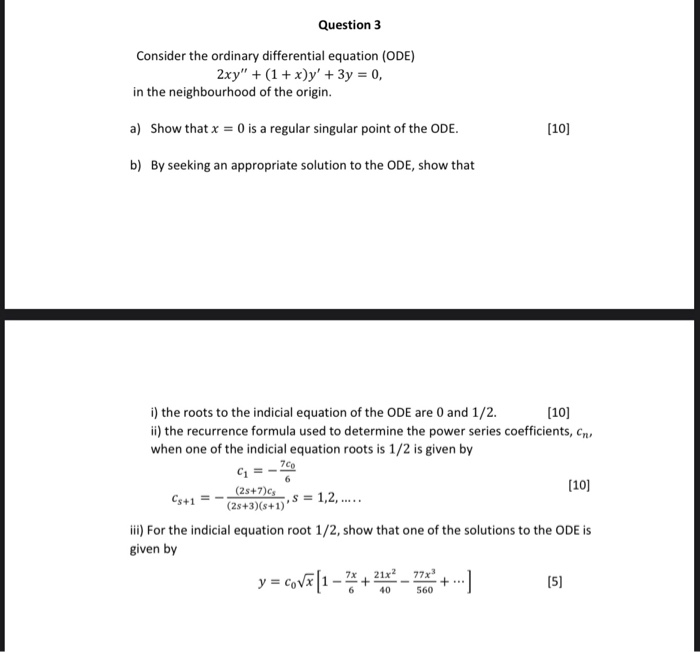Question 3 Consider the ordinary differential equation (ODE) 2xy" + (1 + x)y' + 3y = 0, in the neighbourhood of the origin. a) Show that x = 0 is a regular singular point of the ODE. (10) b) By seeking an appropriate solution to the ODE, show that G=- (10) i) the roots to the indicial equation of the ODE are 0 and 1/2.  ii) the recurrence formula used to determine the power series coefficients, ens when one...

• ### Need some help with SERIES SOLUTION - 2nd ORDER EQUATION For the differential equation,          (1) a. Calculate the indicial equation for the power series solution (Answer in a quadratic polynomial...

Need some help with SERIES SOLUTION - 2nd ORDER EQUATION For the differential equation,          (1) a. Calculate the indicial equation for the power series solution (Answer in a quadratic polynomial in terms of c.) b. Calculate the solutions of the indicial equation found above. c. Calculate the point from the above equation (1) as i. ORDINARY POINT ii. REGULAR SINGULAR POINT iii. IRREGULAR SINGULAR POINT We were unable to transcribe this imagey-Σ@m(z _ 4)nte We were unable to transcribe this...

• ### Differential Equations: Please read carefully and choose the best answer 1.1 1.2 1.3 1.4 In the...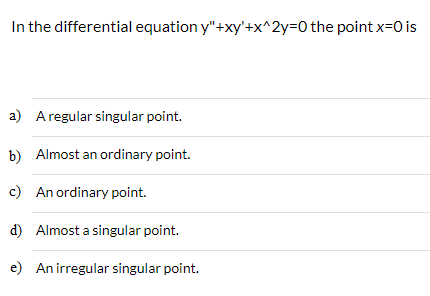Differential Equations: Please read carefully and choose the best answer 1.1 1.2 1.3 1.4 In the differential equation y"+xy'+x^2y 0 the point x 0 is a) Aregular singular point. Almost an ordinary point. b) c) An ordinary point. d) Almost a singular point. e) An irregular singular point. Which of the following cannot be an interval of convergence for a power series: a) -1<x>1 b) x0 c) 0<x<+infinity d) infinity<x<+infinity e) -1<=x<-1 A power series solution of a differential equation...

• ### Find two power series solutions of the given differential equation about the ordinary point x =...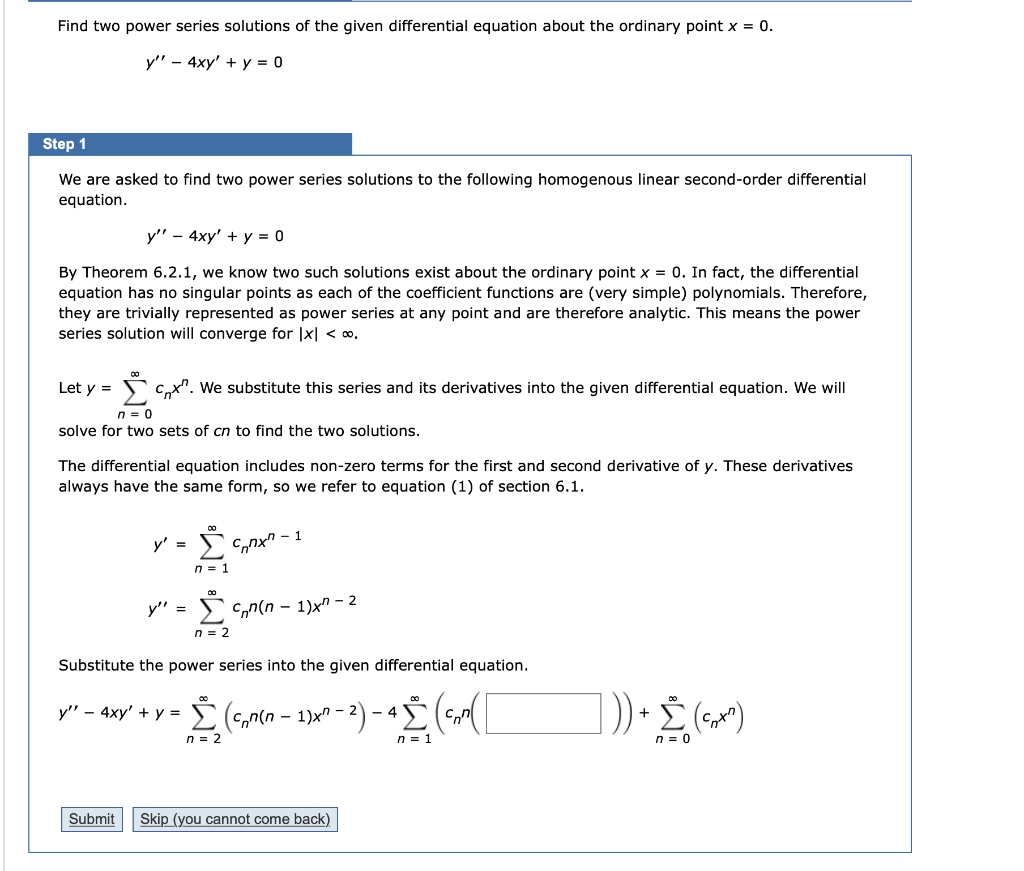Find two power series solutions of the given differential equation about the ordinary point x = 0. y′′ − 4xy′ + y = 0 Find two power series solutions of the given differential equation about the ordinary point x = 0. y!' - 4xy' + y = 0 Step 1 We are asked to find two power series solutions to the following homogenous linear second-order differential equation. y" - 4xy' + y = 0 By Theorem 6.2.1, we know two...

• ### Consider the differential equation 4x2y′′ − 8x2y′ + (4x2 + 1)y = 0 (a) Verify that x0 = 0 is a regular singular point of...

Consider the differential equation 4x2y′′ − 8x2y′ + (4x2 + 1)y = 0 (a) Verify that x0 = 0 is a regular singular point of the differential equation and then find one solution as a Frobenius series centered at x0 = 0. The indicial equation has a single root with multiplicity two. Therefore the differential equation has only one Frobenius series solution. Write your solution in terms of familiar elementary functions. (b) Use Reduction of Order to find a second...

Free Homework App

Scan Your Homework
to Get Instant Free Answers
Need Online Homework Help?

Get Answers For Free
Most questions answered within 3 hours.